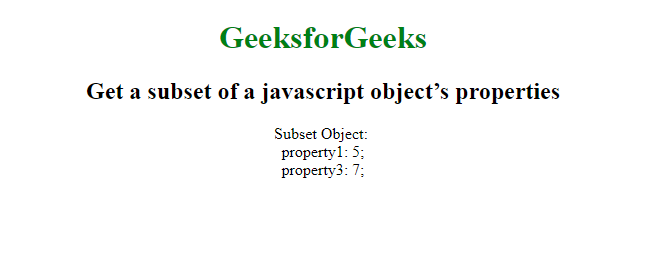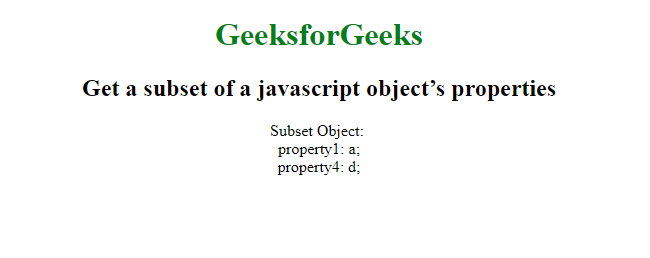# How to get a subset of a javascript object’s properties?

To get the subset of properties of a JavaScript Object, we make use of destructuring and Property Shorthand. The destructuring assignment syntax is a JavaScript expression that makes it possible to unpack values from arrays, or properties from objects, into distinct variables.
Syntax:

`subset = (({a, c}) => ({a, c}))(obj);`

Example1: Get subset of a javascript object’s properties using destructuring assignment.

## html

 `` `<``html``>`   `<``head``>` `    ``<``title``>` `      ``Get a subset of a javascript object’s properties` `  ``` ``   `<``body``>` `    ``<``center``>` `        ``<``h1` `style``=``"color:green"``>` `          ``GeeksforGeeks` `      ``` `        ``<``h2``>` `          ``Get a subset of a javascript object’s properties` `      ``` `        ``<``script``>` `            ``obj = {` `                ``property1: 5,` `                ``property2: 6,` `                ``property3: 7` `            ``};` `            ``subset = (({` `                ``property1, property3` `            ``}) => ({` `                ``property1, property3` `            ``}))(obj);`   `            ``var output = 'Subset Object: <``br``>';` `            ``for (var property in subset) {` `                ``output += property + ': ' + subset[property] + ';<``br``>';` `            ``}` `            ``document.write(output);` `        ``` `    ``` ``   ``

Output:In ES6 there is a very concise way to do this using destructing. Destructing allows you to easily add on to objects and make subset objects.
Syntax:

```const {c, d, ...partialObject} = object;
const subset = {c, d};```

Example 2: Get subset of a javascript object’s properties using destructuring assignment.

## html

 `` `<``html``>`   `<``head``>` `    ``<``title``>` `      ``Get a subset of a javascript object’s properties` `  ``` ``   `<``body``>` `    ``<``center``>` `        ``<``h1` `style``=``"color:green"``>` `          ``GeeksforGeeks` `      ``` `        ``<``h2``>` `          ``Get a subset of a javascript object’s properties` `      ``` `        ``<``script``>` `            ``const object = {` `                ``property1: 'a',` `                ``property2: 'b',` `                ``property3: 'c',` `                ``property4: 'd',` `            ``}`   `            ``const {` `                ``property1,` `                ``property4,` `                ``...pObject` `            ``}`   `            ``= object;` `            ``const subset = {` `                ``property1,` `                ``property4` `            ``}`   `            ``;` `            ``var output = 'Subset Object: <``br``>';` `            ``for (var property in subset) {` `                ``output += property + ': ' +` `                    ``subset[property] + ';<``br``>';` `            ``}`   `            ``document.write(output);` `        ``` `    ``` ``   ``

Output:Whether you're preparing for your first job interview or aiming to upskill in this ever-evolving tech landscape, GeeksforGeeks Courses are your key to success. We provide top-quality content at affordable prices, all geared towards accelerating your growth in a time-bound manner. Join the millions we've already empowered, and we're here to do the same for you. Don't miss out - check it out now!

Previous
Next